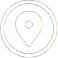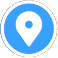### Virtual Tour

Start Virtual TourLaunch Virtual TourStart Virtual Tour

### Placement Tests in Mathematics

#### ACCUPLACER Test

This test consists of two parts: ALGEbra and ARIThmetic. Students who would like to take a general education mathematics course such as MATH 101, MATH 104, MATH 106, or MATH 108, are required to take the ACCUPLACER test, except in the instances listed in the remaining portion of this paragraph. Students whose math portion of SAT test is 560 or above, or whose ACT composite score is 26 or above, are exempt from this test and may register for their general education math course. Students whose math portion of SAT test is 530 to 550, are only required to take the ALGEbra portion of the ACCUPLACER test, and do not need to take the ARIThmetic portion of the test. Students may not register for their general education mathematics course without completing the mathematics course(s) indicated by the ACCUPLACER test, if the test indicates such.

#### CLM Test

Students who would like to take Calculus I (MATH 121) or Discrete Structures (MATH 237), (for instance: science majors or mathematics majors), are required to take the College Level Math test  (i.e. the CLM test), unless they successfully completed the prerequisite course (college level Precalculus, or formerly Precalculus II). Students whose math portion of SAT test is 560 or above (or whose ACT composite score is 26 or above), may register to take the CLM test without any additional testing. Students whose math portion of SAT test is below 560, must achieve 82 or higher on the ALGEbra portion of the ACCUPLACER in order to register for the CLM test. Students who successfully completed college-level Precalculus (formerly Precalculus II) may register for Calculus I or Discrete Structures without taking the CLM test. Students who successfully completed Calculus I may register for Discrete Structures without testing. Students may not register for Calculus I or Discrete Structures without completing the mathematics course(s) indicated by the CLM test, if the test indicates such.

for details of how to register to take a placement test, to see practice tests, to see which courses you may place into depending on the results of your test, to see test exemptions, etc.

### AP Credits in Mathematics

• At Ramapo College, students with a score of 4 or 5 on AP Calculus AB test receive credits for Calculus I (MATH 121).
• Students with a score of 4 or 5 on AP Calculus BC test receive credits for Calculus I (MATH 121) and Calculus II (MATH 122).
• Students with a score of 3 on AP Calculus AB test are exempt from taking the CLM placement test and can register for Calculus I or Discrete Structures after asking the course instructor to sign them into the course and showing a proper score documentation.
• At Ramapo College, students with a score of 4 or 5 on AP Statistics test receive credits for Elementary Probability and Statistics (MATH 108).